﻿ Complexity Analysis of Double-Threshold Based Relay Selection in D2D Cooperative NetworkJournal of Wireless Networking and Communications

p-ISSN: 2167-7328    e-ISSN: 2167-7336

2018;  8(1): 1-6

doi:10.5923/j.jwnc.20180801.01### Complexity Analysis of Double-Threshold Based Relay Selection in D2D Cooperative Network

Shamganth Kumarapandian1, Martin James Sibley2

1Engineering Department, Ibra College of Technology, Ibra, Oman

2School of Engineering and Computing, University of Huddersfield, Huddersfield, United Kingdom

Correspondence to: Shamganth Kumarapandian, Engineering Department, Ibra College of Technology, Ibra, Oman.
 Email: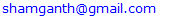Abstract

In this paper, a novel dual-threshold based relay selection scheme and combining scheme for dual-hop differential amplify and forward cooperative D2D cooperative Cellular networks is proposed. Also, a novel combining scheme, Dual-Threshold MRC (DT-MRC) with differential AF modulation is proposed. Analysis of the number of path estimation and active branches is very much essential in order to determine the system complexity. Also the active branch determination provides the power savings of the proposed scheme as compared to the existing schemes. Numerical results show that the proposed scheme has a reduction in the average number of path estimation.

Keywords: Cooperative Wireless network, D2D, Relay selection, Differential Amplify and Forward

Cite this paper: Shamganth Kumarapandian, Martin James Sibley, Complexity Analysis of Double-Threshold Based Relay Selection in D2D Cooperative Network, Journal of Wireless Networking and Communications, Vol. 8 No. 1, 2018, pp. 1-6. doi: 10.5923/j.jwnc.20180801.01.

### 1. Introduction

Device-to-Device (D2D) communication is considered as a promising technique in 5G cellular network technologies. D2D communication in cellular network refers to the direct communication between the mobile users without the involvement of Base Station (BS) or the core network elements.
Combining schemes classified into two main types: Input threshold schemes and output threshold schemes. Output threshold schemes such as OT-MRC  and MS-GSC reduce power consumption, but it may select low SNR branches. Input threshold schemes such as AT-GSC and NT-GSC  has the number of relays chosen, and hence it has more complexity.
In this paper, a novel relay selection scheme is proposed. And the proposed technique is based on distributed multi-relay selection. Proposed relay selection scheme combines the merits of threshold-based relay selection , double-threshold relay selection  and relay selection with feedback .

### 2. Prior Related Research

Furuzan Atay Onat et al.  proposed relay selection cooperation protocol. The relays with the received SNR are larger than a threshold is considered as reliable relays, and it’s allowed to retransmit. This protocol employs selection combing at the destination.
Hong-Chuan Yang et al.  proposed the output threshold MRC (OT-MRC) scheme. In this scheme, the receiver adaptively combines as many diversity paths as necessary to have the output SNR exceed a preselected threshold. And extend the idea of output threshold of GSC scheme to reduce its processing power.
Gayan Amarasuriya et al.  proposed the output threshold multi-relay selection scheme (OT-MRS) which uses orthogonal channeling and distributed AF relays with MRC combining at the destination.
Athanasios S. Lioumpas et al.  combines the features of MS-GSC and NT-GSC in their proposed Adaptive Generalized Selection combining (AT-GSC). And this scheme achieves better adaption to channel conditions without increasing the system complexity.
Ning Xie et al.  proposed a diversity combining scheme based on generalized selection combining with double threshold. In this scheme, two thresholds were set, and the relay is selected if the SNR of the source to relay branch exceeds the input threshold and the process continues until the combined SNR exceeds the output threshold.
M. Balasaraswathi et al.  proposed threshold based selection combining for relay networks. DF based relays used in this work and its shown detection errors can be minimized by setting a threshold at the relay.
M. Dayanidhy et al.  proposed the new form of selection combining for multiple intermediate nodes using full CSI.

### 3. Motivation and our Contribution

Single relay selection schemes suffer from performance loss regarding error rate and outage probability. The complexity of the optimal Multi-relay selection schemes  increases exponentially with the available number of relays. Generalized section combining (GSC) based MRS schemes achieves performance gains, it requires the channel estimation of all the relayed paths. Also, GSC based MRS schemes select more relays than required. This problem is overcome by GSC with the double threshold (DT-GSC) . In all the prior works the full channel estimation is required which is not suitable for the practical conditions. Also in the earlier works to overcome this problem we extend the idea of OT-MRC  and multi-hop threshold relay selection scheme  by setting the threshold at the relay node as input threshold and the destination node as output threshold and proposed Double Threshold MRC (DT-MRC) scheme with differential modulation. Acquiring CSI in fast fading environment is not practical. In differential modulation for example DPSK relies only on the constant phase response of the channel and perfect CSI is not required. Differential modulation considered to be the promising candidate since it reduces the receiver complexity and signal overheads bypassing channel estimation. In  the DiffAF scheme requires only long term average of the received signals and no instantaneous CSI is required. In this scheme input threshold is set based on the signal quality per branch and output threshold is based on the signal quality at the combiner output.

### 4. Categories of D2D Communication

D2D communication is categorized into two types as Inband and Outband communication. This study relates to the outbound mode of D2D which is classified into two categories as Controlled and Autonomous outband modes. Outband D2D communication uses the unlicensed spectrum. In Autonomous D2D mode communication devices perform the network setup as shown in Figure 1. The proposed relay selection scheme can be implemented in autonomous D2D mode.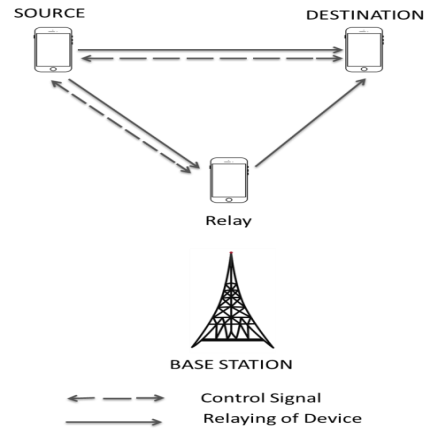Figure 1. Device relaying with full control from device 

### 5. Proposed Relay Selection Scheme

In this study, a double threshold based relay selection technique with MRC is presented. The difference between the proposed relay selection technique and threshold-based relay selection in [2, 13] is single threshold used at the relay. In the proposed method Differential AF (DiffAF) scheme is used which does not require the full CSI at the relays and the destination . So the complexity reduction can be achieved by the proposed scheme.
Source sends the signal to the destination, and the relays in the coverage region will also receive during the broadcast phase. If the received SNR at the relays is higher than the threshold SNR then will be in the listening mode, and the remaining relays will be sleeping mode. Destination will provide the feedback to the source based on the combined SNR of the relayed path and direct path SNR. If the combined SNR falls below the threshold value, then NACK will be sent by the destination to the source for source retransmission. Figure.3 shows the mode of operation of the proposed scheme.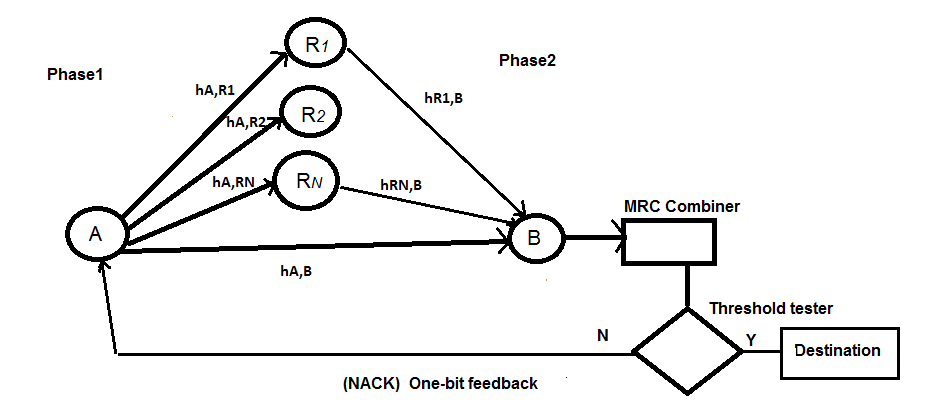Figure 2. System model

### 6. System Model

Cooperative wireless network with one source N-1 relays and one destination node is shown in the system model. Multi-branch dual-hop relaying with direct link is considered in this model in Figure 2. It has a total of 2N-1 fading channels that connect source, relays and destination if all the relays satisfies the input threshold condition. The terminals includes the source node A, destination node B and N-Differential AF relay nodes in an uncoded system. It is assumed that all the nodes use the single antenna. Multinode cooperative wireless is considered in this model.
The transmission process is divided into two phases :
Phase1: In the first phase (i.e. broadcast phase) node ‘A' transmits the information bits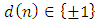(1)
at A are differentially encoded.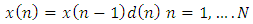(2)
In equ(2), x(n) is the signal transmitted from A. And ‘N’ is the number of bits in one frame. Signals received at the relays R and destination B is,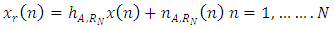(3)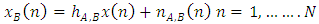(4)
In equ(3) and (4),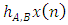and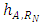are the fading coefficients of the channels Source A to relays RN and Relays to destination.
The channels A-RN and RN - B are independent and identically distributed and undergo Rayleigh flat fading. Also, the direct path from source A to destination B is considered as Rayleigh fading channel in the model.
Phase2: In the second phase (i.e., relaying phase) relay selection criteria are applied i.e., only (1≤Ni≤N) relays out of N relays are selected. It is based on the condition if the source to relay SNR (A-RN) exceeds the input threshold (γiT) then the relays are chosen to forward the source transmission by the amplify and forward (AF) technique.
In phase2, the relay amplifies the signal from the source and forwards it to the destination ideally equalizes the effect of the channel fade between the source and relay. The received signal at the relay is amplified and retransmitted to the destination.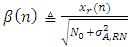(5)
In equ(5),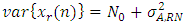can be estimated by time-averaging over a frame of received signal.
The received signal at B from the relay Ri is written as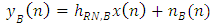(6)
For differential detection, fading channels are assumed to be static over a finite time interval.
The instantaneous SNR between the nodes is given as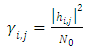(7)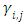is exponentially distributed with PDF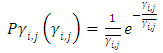(8)
Differential detection at destination B is given as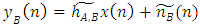(9)
Where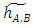is the effective channel gain of the relay link (A-RN-B) defined as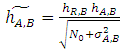(10)
Where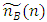is the effective channel noise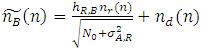(11)
If the direct link SNR is above the output threshold (γOT) then differential detection  in this case using (2) and (4) we have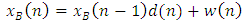(12)
Where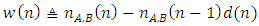Using (2) and (9) we have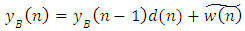(13)
Where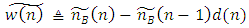Applying joint pdf of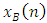and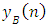the differential combiner  as given as: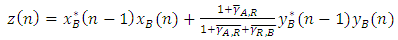(14)
Where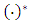is the complex conjugation. And (14) has different weights for different branches. Also in the differential combiner we have average SNR for (A-R) and (R-B) links which differs from the standard linear combiner by avoiding the instantaneous SNR.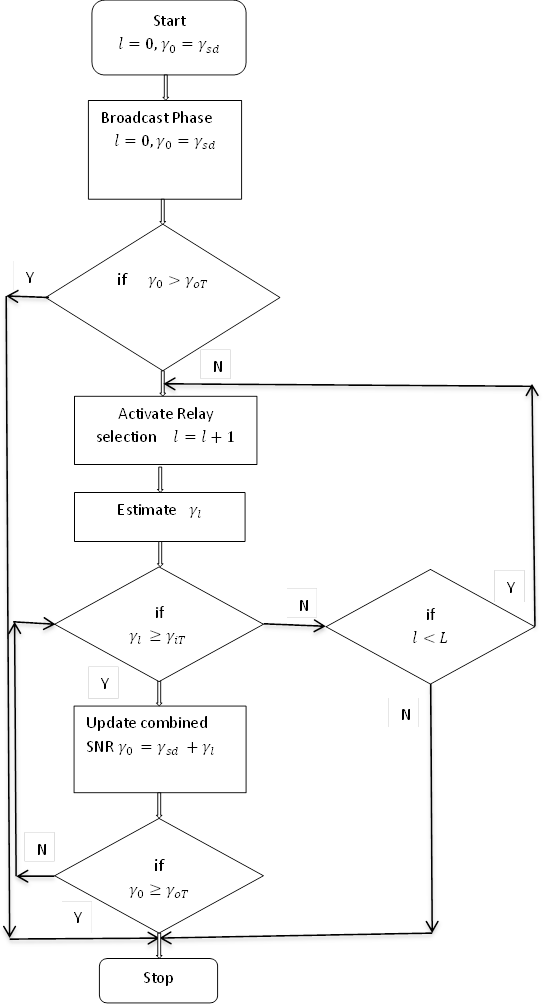Figure 3. Mode of Operation

### 7. Performance Analysis

To analyse the performance of the proposed relay selection scheme, statistical characterization is needed. In this section the cumulative distribution function (cdf), probability density function (pdf) and the moment generating function (MGF) are derived for the combined SNR of the proposed relay selection scheme.
The instantaneous output SNR (γ0) of the proposed scheme can be written as follows: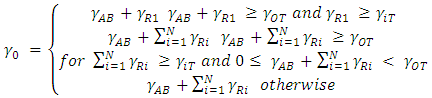(15)
where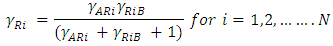is the relayed path of the ith active relays for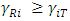.
General expression for the cdf of the output SNR can be written as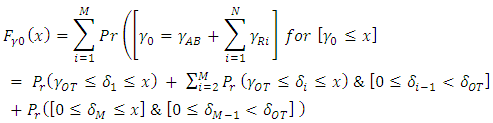(16)
In equation (16), δi is the combined SNR of the first ‘i’ relayed paths and direct path it is given as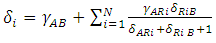(17)
In equation (16) the first term refers to the combined SNR of the direct path and the first relayed path is above the output threshold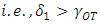The second term is based on ‘i’ relays combined with the direct path to form an output SNR above the threshold (γOT).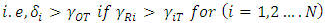.
The third term is the worst case where the sum of N-1 relayed paths and the direct path does not exceed the output threshold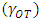.
From the mode of operation of the proposed scheme, an observation is listed below:
1. A single relay forwards the source information, and the relayed signal is combined with the direct path in an MRC combiner if the SNR at the relay is above input threshold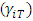and the combined SNR is above the output threshold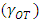.
2. An ith branch MRC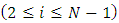is used when the combined SNR of an N-1 branch and the direct path is above the output threshold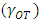.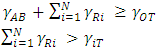3. No relay is selected, and feedback is sent to the source to retransmit the data. i.e.,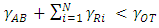Mathematically: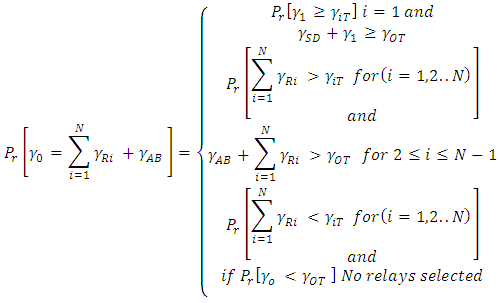(18)
Applying total probability theorem, the cdf of the output SNR P0(x) as,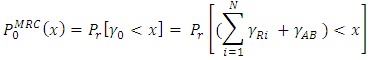(19)
Let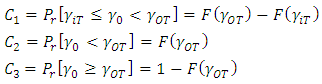(20)
Average channel estimation
Increase in channel estimation has an increase in the complexity
Case (i): Ne =1; in this case, the first branch SNR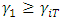and the combined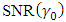where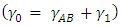is greater than output threshold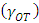, i.e.,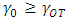. So the probability can be written as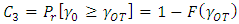(21)
Case (ii): If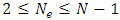there are two cases:
a. The first N-1 branches SNR is below the input threshold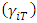, but the relay sum of ith relay SNR and the direct path is above the output threshold.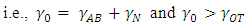.
The probability is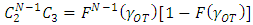(21a)
b. From the relayed path, which is above the input threshold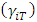, the combined SNR of the first N-1 branch SNR and the direct path SNR is below the output threshold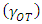,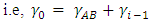for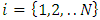and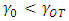but the combined SNR of the Nth branch and the direct path is above the output threshold.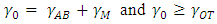The probability is given by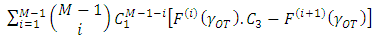(22)
For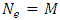; the probability is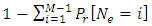After arranging, the number of path estimation calculated as follows: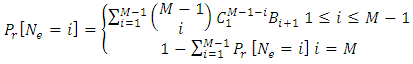(23)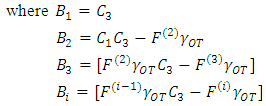Mathematical expression for average number of path estimation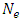is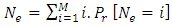(24)
Average number of combined branches
Based on the mode of operation of the proposed scheme we observe the following:
1.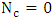; all the branch SNR is less than input threshold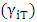and the combined SNR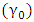is less than output threshold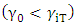. The probability is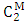in this case first M-1 branches SNR is below input threshold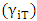, so it is in the sleep mode and the Mth branch SNR is above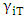and the combined branch SNR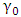is above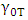.
2.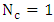;
Case (i): only one of the branch SNR is above input threshold, and the combined SNR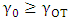probability is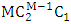Case (ii): In this case first M-1 branch SNR is below input threshold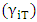, so it’s in sleep mode and the Mth branch SNR is above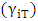and the combined branch
SNR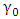is above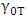. So the probability is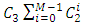Probability of combining one branch is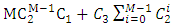(25)
3.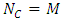; all the branch SNR is above input threshold, and the combined SNR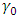is above output threshold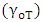. The probability is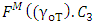.

### 8. List of Abbreviations

D2D - Device to Device
DT-MRC – Double Threshold Maximum Ratio Combining
OT-MRC - Output Threshold Maximum Ratio Combining
A-GSC – Adaptive Generalized Selection Combining
BS- Base Station
MS-GSC - Minimum Selection Generalized Selection Combining
NT-GSC - Normalized Threshold Generalized Selection Combining
OT-MRS - Output Threshold Multi-Relay Selection
AT-GSC - Adaptive Threshold Generalized Selection Combining
CSI - Channel State Information
GSC - Generalized Selection Combining
MRC - Maximum Ratio Combining
AF - Amplify and Forward
DF - Decode and Forward
SNR- Signal to Noise Ratio

### 9. Numerical Results

In this section numerical results are presented for average number of channel estimation. And average channel estimation is analyzed in different cases as shown below:
Case(i): For Ne=1 only for the first branch channel estimation is required, since the first branch SNR is greater than the input (γiT ) and the combined SNR(γ0) is greater than output threshold (γOT). For rayleigh fading channel the probability is calculated using (21) for the normalized SNR ranges from -15dB to 15dB. It is evident from the table 1, for high values of Normalized SNR the number of channel estimation is reduced.
 Table 1. Channel estimation for Case (i)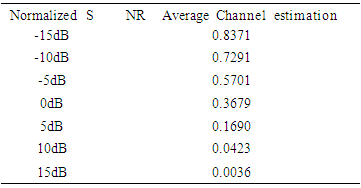Case (ii): For 2 ≤ Ne N − 1 probability is calculated using (21a) as shown in table 2.
 Table 2. Channel estimation for Case (ii)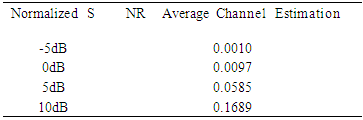### 10. Conclusions

In this paper, we proposed a novel dual-threshold based relay selection with Differential amplify and forward (DiffAF) scheme. Due to the differential modulation used in the instantaneous SNR from each branch is not required so the system complexity is drastically reduced. As we can observe from the numerical results the average channel estimation reduced based on the increase in normalized SNR. We analyzed the average number of path estimation and combined branches of the proposed scheme.

### References

  Furzan Atlay Onat, “Threshold Selection for SNR-based Selective Digital Relaying in Cooperative Wireless Networks,” IEEE Transactions on Wireless Communications, vol.7, No.11, pp.4226-4237, Nov. 2008.  Athanasios S. Lioumpas, George K. Karagiannidis, Theodoros A. Tsiftsis, “Adaptive Generalized Selection Combining (A-GSC) Receivers," IEEE Transactions on Wireless Communications, vol. 7, No. 12, Dec, 2008.  Kuang-Hao Liu, “Double Threshold Digital Relaying for Cooperative Wireless Networks,” IEEE 71st Vehicular Technology Conference (VTC 2010-Spring), 2010.  Bletsas, A., Khisti, A., & Win, M. Z, “Opportunistic cooperative diversity with feedback and cheap radios,” IEEE Transaction on Wireless Communications, pp. 1823–1827, 2007.  Furzan Atlay Onat, "Threshold-based relay selection in Cooperative Wireless Networks," IEEE-GLOBECOM, 2008.  Hong-Chuan Yang, Mohamed-Slim Alouini, “MRC and GSC Diversity Combining,” IEEE Transactions on Vehicular Technology, vol. 54, No.3, May 2005.  Gayan Amarasuriya, Masoud Ardakani, Chintha Tellambura, “Output-Threshold Multiple-Relay-Selection Scheme for Cooperative Wireless Networks,” IEEE Transactions on Vehicular Technology, vol. 59, No. 6, July 2010.  Onat, F. A., Yanikomeroglu, H., Fan, Y., & Poor, H. V, “Threshold-based relay selection for detect and-forward relaying in cooperative wireless networks," EURASIP Journal on wireless communications and networking 2010.  Ning Xie, Zhaorong Ning Xie, Zhaorong Liu, Rui Wang, Hui Wang, Xiaohui Lin, “Generalized selection combining with the double threshold and performance analysis,” Wireless Communications and Mobile Computing, vol.14, pp.56-63, 2014.  V. Nagarajan, M. Balasaraswathi, “Threshold-based selection combining for relay networks,” ELSEVIER, Computers and Electrical Engineering, vol. 60, pp129-139, 2017.  M. Dayanidhy, V. Jawahar Senthil Kumar, “Performance investigation of multi-relay cooperative diversity networks,” ELSEVIER, Computers and Electrical Engineering, vol.60, pp151-160, 2017.  M. O. Hasna, M. S. Alouini, “A Performance Study of Dual-Hop Transmissions with Fixed Gain Relays,” IEEE Transactions on Wireless Communications, vol. 3, December 2004.  K. Shamganth, Dr. Martin Sibley, Dr. Sami Ghnimi “A Novel Multi-hop Threshold based Relay Selection Scheme,” International Journal of Advanced Research in Computer Science and Software Engineering, Vol.4, Issue.4, April 2014.  Pimmy Gandotra, Rakesh Kumar Jha, “Device-to-Device Communication in Cellular Networks: A Survey,” Journal of Network and Computer Applications, vol.71, pp.99-117, 2016.  Arash Asadi, Qing Wang, and Vincenzo Mancuso, “A Survey on Device-to-Device Communication in Cellular Networks,” IEEE Communiction Surveys & Tutorials, vol. 16, No. 4, pp.1801-1819, 2014.  T. Himsoon, W. Su, and K. J. R. Liu, “Differential transmission for amplify-and-forward cooperative communications,” IEEE Signal Process. Lett., vol. 12, no. 9, pp. 597–600, Sept. 2005.  M. R. Avendi, Ha H. Nguyen, “Differential Amplify-and-Forward Relaying in Time-Varying Rayleigh Fading Channels,” IEEE Wireless Communication and Networking Conference (WCNC), 2013.  Qiang Zhao, Hongbin Li, “Differential Modulation for Cooperative Wireless Systems,” IEEE Transactions on Signal Processing, vo. 55, No. 5, May 2007.